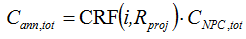# HOMER Pro 3.9

 Navigation: Glossary Total Annualized CostType: Output Variable Units: \$/year Symbol: Cann,tot

The total annualized cost is the annualized value of the total net present cost. HOMER calculates the total annualized cost using the following equation:where: CNPC,tot = the total net present cost [\$] i = the annual real discount rate [%] Rproj = the project lifetime [yr] CRF() = a function returning the capital recovery factor

HOMER uses the total annualized cost to calculate the levelized cost of energy.

See also

Annualized Cost

Total Net Present Cost

Levelized Cost of Energy